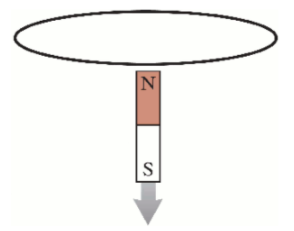# Problem: In the first question, we determined the direction of the induced current in the conducting loop as the magnet was approaching it from above, south pole first. Now imagine the magnet has gone through the loop and is now below the loop, falling away from it (still south pole first). (Figure 1) Part A. Determine the direction of the magnetic field of the magnet through the loop.A. upwardB. downwardPart B. As the magnet falls away from the loop, how is the magnetic flux through the loop changing?A. It is increasing.B. It is decreasing.C. There is no change in the flux.Part C. According to Lenz's law, in which direction is the magnetic field induced by the loop?A. upwardB. downward

###### FREE Expert Solution

Part A

Magnetic field lines originate from the north pole and are directed into the south pole.

98% (435 ratings)###### Problem Details

In the first question, we determined the direction of the induced current in the conducting loop as the magnet was approaching it from above, south pole first. Now imagine the magnet has gone through the loop and is now below the loop, falling away from it (still south pole first). (Figure 1)Part A. Determine the direction of the magnetic field of the magnet through the loop.

A. upward
B. downward

Part B. As the magnet falls away from the loop, how is the magnetic flux through the loop changing?

A. It is increasing.
B. It is decreasing.
C. There is no change in the flux.

Part C. According to Lenz's law, in which direction is the magnetic field induced by the loop?

A. upward
B. downward